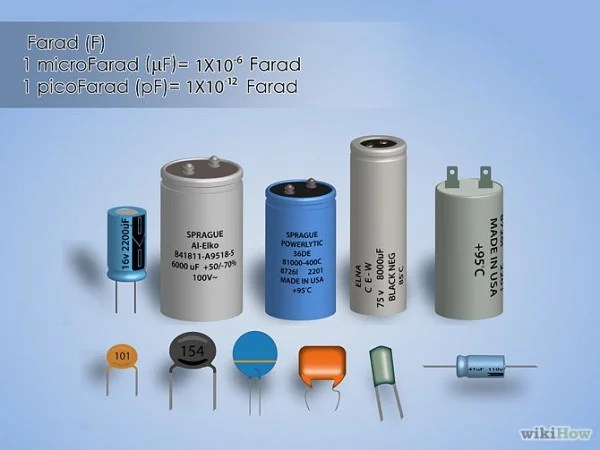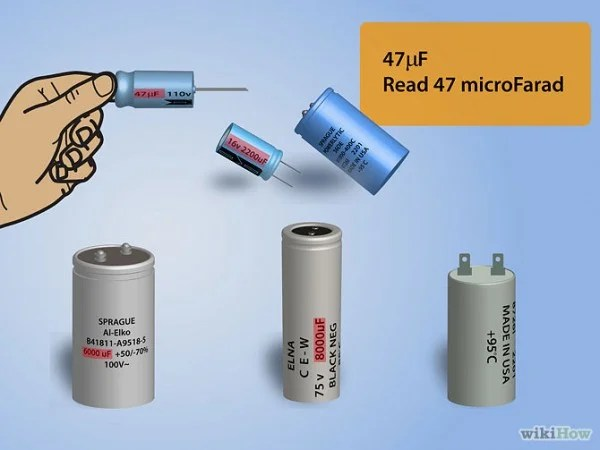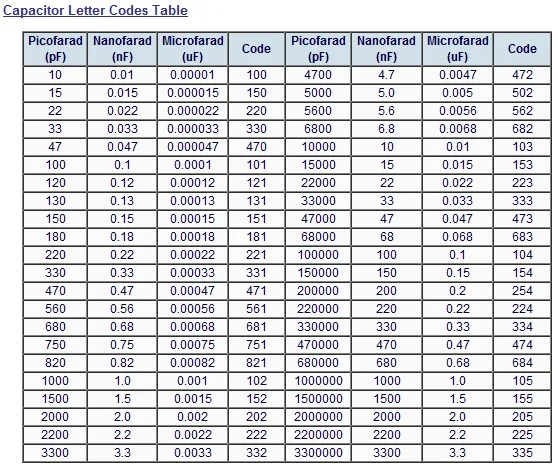# How to read capacitor code

Capacitors are elements of a circuit that react to rapidly changing signals, rather than slowly changing or static signals. Capacitors can store the energy from strong rapidly changing signals and return that energy to the circuit as desired. The most common usage of capacitors is to absorb noise, which is by definition a rapidly changing signal, and divert it away from the signal of interest. Different capacitor values are needed to trap different types of noise. Use these tips to learn how to read capacitor designations and determine the value of the capacitor.## STEP 1

Understand the units of measurement used for capacitors. The base unit of capacitance is the Farad (F). This value is too large to be of use in a circuit. Smaller denominations of capacitance are used by electronic circuits.## STEP 2

Read the value directly on larger bodied capacitors. If the surface of the body is large enough, the value will be printed directly on the capacitor. For example, 47 uF indicates 47 microFarads.## STEP 3:

Read the capacitance of smaller bodied capacitors as two or three numbers.The designators uF or pF will not appear due to the small size of the capacitor body.

• Read two digit numbers as being in picoFarads (pF). For example, 47 would be read as 47 pF.
• Read three digit numbers as a base capacitance value in picoFarads and a multiplier. The first two digits will indicate the base capacitor value in picoFarads. The third digit will indicate a multiplier to be used on the base number to find the actual value of the capacitor.
• Use a third digit of 0 through 5 to place the corresponding number of 0s behind the base value. A third digit of 8 means multiply the base value by .01. A third digit of 9 means to multiply the base value by 0.1. For example, 472 would indicate a 4700 pF capacitor and 479 would indicate a 4.7 pF capacitor.
• Digit-Character-Digit. Some small capacitors are marked with codes like 1n0. The digits are the values before and after the decimal point and the the character tells you the dimension; so the example given is 1.0 nF (nano-Farad).## STEP 4:

Look for a letter code. Some capacitors are defined by a three number code followed by a letter. This letter represents the tolerance of the capacitor, meaning how close the actual value of the capacitor can be expected to be to the indicated value of the capacitor. The tolerances are indicated as follows.

• Read B as 0.10 percent.
• Read C as 0.25 percent.
• Read D as 0.5 percent.
• Read E as 0.5 percent. This is a duplication of a D code.
• Read F as 1 percent.
• Read G as 2 percent.
• Read H as 3 percent.
• Read J as 5 percent.
• Read K as 10 percent.
• Read M as 20 percent.
• Read N as 0.05 percent.
• Read P as plus 100 percent to minus 0 percent.
• Read Z as plus 80 percent to minus 20 percent.

ELECTROLYTIC CAPACITOR

An electrolytic capacitor is a polarized capacitor which uses an electrolyte to achieve a larger capacitance than other capacitor types.In the case of through-hole capacitors, the capacitance value as well as the maximum rated voltage is printed on the enclosure. A capacitor that has “4.7μF 25V“ printed on it has a nominal capacitance value of 4.7μF and a maximum voltage rating of 25 volts, which is never to be exceeded.

In the case of SMD (surface mounted) electrolytic capacitors, there are two basic marking types. The first one clearly states the value in microfarads and the operating voltage. For example, using this approach, a 4.7 μF capacitor with an operating voltage of 25 volts would bear the marking “4.7 25V”. In the other marking system, a letter is followed by three numbers. The letter represents the voltage rating according to the table below. The first two numbers represent the value in picofarads, while the third number is the number of zeroes to be added to the first two. For example, a 4.7 μF capacitor with a voltage rating of 25 volts would bear the marking E476. This translates to 47000000 pF = 47000 nF = 47 μF.

 Letter Voltage e 2.5 G 4 J 6.3 A 10 C 16 D 20 E 25 V 35 H 50

You can read the rest at http://www.capacitorguide.com/electrolytic-capacitor/